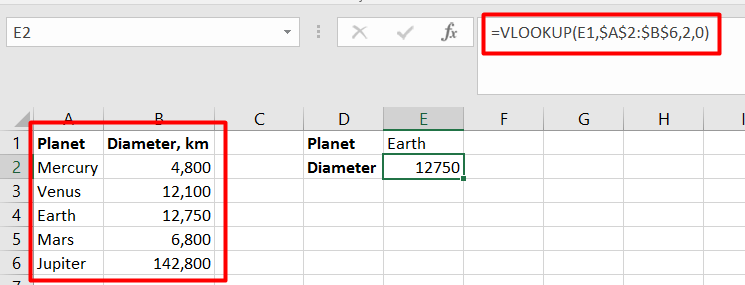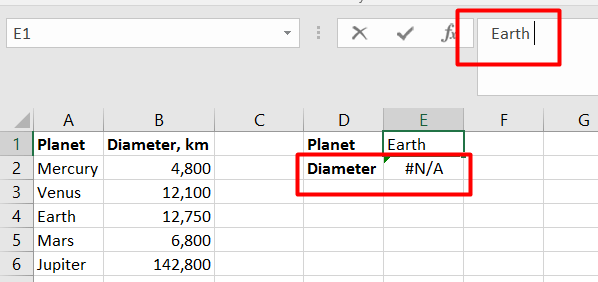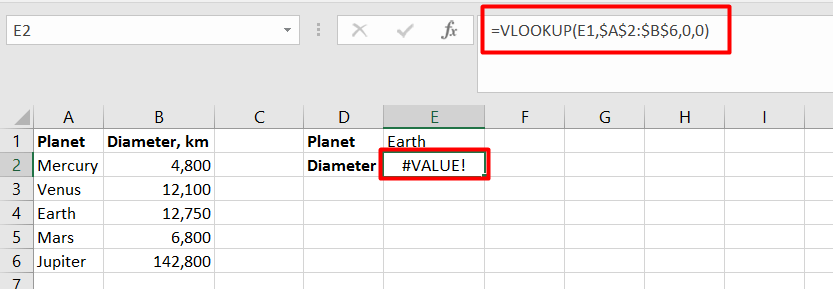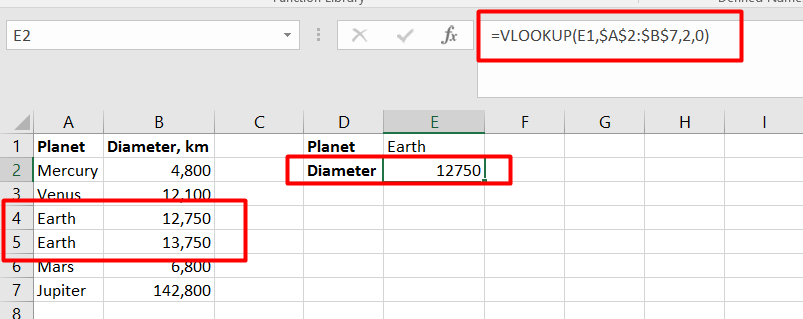# How to Fix VLOOKUP Errors

## How to Fix VLOOKUP Errors

Summary:

VLOOKUP function in Excel is the most widely used function and it comes in very handy while looking up the value from different data sources. However, VLOOKUP also has a lot of limitations and specificities, which leads to various problems and errors.

In this article, we are going to discuss different use cases when VLOOKUP returns #N/A and #VALUE errors. Try improving your Excel skills with  Excel Beginner to Advanced Course

### “#N/A” error in VLOOKUP:

VLOOKUP returns #N/A error when excel cannot find a lookup value. There can be multiple reasons for that:

1. #N/A error in exact match VLOOKUP: As mentioned above, the most of the obvious reason of getting this error is when lookup value is not present in the lookup array. The user needs to make sure that “lookup array” must contain the “lookup value”
2. Lookup value entered contains typo or misprint: There can be server instances where the user typed lookup value directly in the formula. In that situation, it may possible that typed lookup value contains some typo. Therefore, it is always a good idea to check lookup value first when the user gets “#N/A” error
3. The Lookup Column in the Lookup Array: The major limitation of LOOKUP function in Excel is that it cannot look up the value to its left. It means, the “Lookup Column” should always be the left-most column of “Lookup Array”

### Correct Method:1. Extra spaces either in Lookup value or Lookup array: Another source of “#N/A” error is due to extra spaces present in either “Lookup Value” or “Lookup Array”. As VLOOKUP function is case-insensitive, any extra space present in the lookup name considered as a part of the text and thus results in “#N/A” error.

Below is the case where there is extra space in “Lookup Value” and therefore the output is “#N/A” error:### “#VALUE” error in VLOOKUP:

In general, the user gets “#VLAUE” error when the data type of the value used in the formula is wrong. In VLOOKUP users get “#VLAUE” error in following cases:

1. The number of characters exceeds to 255 in Lookup Value:

Another limitation of using Excel VLOOKUP function is, it returns “#VALUE” error if “LOOKUP VALUE” contains 256 characters or more.

1. “col_index_num” argument value less than 1: If the user enters the value of “col_index_num” argument value less than 1 in that case as well VLOOKUP functions returns “#VALUE” error as shown below:### Other Limitations of VLOOKUP:

1. VLOOKUP return first found value: In case there are duplicates values in the “Lookup Array”, Excel VLOOKUP function returns the first value it finds in the return column that matches the lookup value1. Adding or deleting a column from the table: Another limitation of VLOOKUP function is, it stops working whenever a new column is added to or deleted from the “Lookup Table”. This happens because in the VLOOKUP function, the user needs to provide table array as well as column number, the value of which needs to be returned. Naturally, both the table array and the return column’s number change if we remove an existing column or insert a new one.
• in
• |
• October 14, 2018#### Gaelim Holland

Subscribe
Notify of